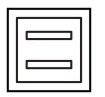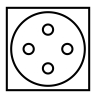0
1488

# Paper Folding Questions for SSC CGL PDF

Download SSC CGL Paper folding questions PDF. Top 5 SSC CGL questions based on asked questions in previous exam papers very important for the SSC exam.

Question 1: A square piece of paper is folded in a particular manner and punched and then unfolded. The unfolded paper is given below. Find out the manner in which the paper was folded and punched from the answer figure.

a)

b)

c)

d)

Question 2: A piece of paper is folded and punched as shown below in the question figures. From the given answer figures, indicate how it will appear when opened.

a)

b)

c)

d)

Question 3: A piece of paper is folded and punched as shown below in the question figures. From the given answer figures, indicate how it will appear when opened.

a)

b)

c)

d)

Question 4: A piece of paper is folded and punched as shown below in the question figures. From the given answer figures, indicate how it will appear when opened.

a)

b)

c)

d)

Question 5: A piece of paper is folded and punched as shown below in the question figures. From the given answer figures, indicate how it will appear when opened?

a)

b)

c)

d)

If the paper is folded and punched as shown in option A, then the resulting unfolded paper would look like the one given in the question.

Hence, option A is the correct answer.

When the paper is unfolded once, the two semi circles will appear at the top as well as bottom, and when the paper is unfolded second time, the circular patterns will appear on the right side as well, the same it appeared on the left.

=> Ans – (C)

When the paper is unfolded the first time, the complete rectangle will appear at the bottom and when the paper is completely unfolded, another rectangle will appear at the top like=> Ans – (C)

Since the cuts are made in the quadrant, when the paper is unfolded the first time, the complete circle will appear at the bottom and the two semi circle will appear at horizontal diagonal and when the paper is completely unfolded, the four circles will appear like=> Ans – (B)